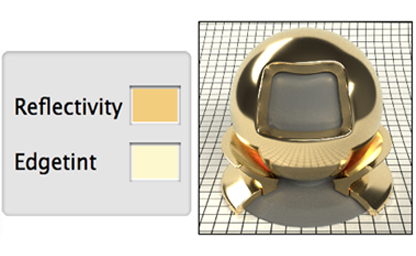# Fresnel

• an conclusion of some resources of fresnel in rendering

# Basics

The interaction of light with a planar interface between two substance follows the Fresnal Equations. The surface is assumed to not have any irregulariies between 1 light wavelength and 100 wavelengths in size.

ight incident on a flat surface split into reflected part and refrated part, we define the angle between surface normal and reflected light direction is $\theta_i$, the angle between surface normal and refracted light direction is $\theta_t$, the Index of Refraction(IOR) of two substance is $n_{i}$, $n_{t}$ (incident, transmitted).

the formula of IOR is $n = \eta + ik$, for dieletric, kappa is zero, we use(light is an electromagnetic wave, and the electric field of this wave oscillates perpendicularly to the direction of propagation)

S polarization is pependicular to the plance of incidence P polarization is parallel to the plance of incidenceWe don’t derive the Fresnal Equation but just give it out. you can find the derivation here

the reflection and refraction coefficient of S polarization and P polarization

$R_s = (\frac{n_i\cos\theta_i-n_t\cos\theta_t}{n_i\cos\theta_i+n_t\cos\theta_t})^2$ $T_s = (\frac{2n_i\cos\theta_i}{n_i\cos\theta_i+n_t\cos\theta_t})^2$

$R_p = (\frac{n_i\cos\theta_t-n_t\cos\theta_i}{n_i\cos\theta_t+n_t\cos\theta_i})^2$ $T_p = (\frac{2n_i\cos\theta_i}{n_i\cos\theta_t+n_t\cos\theta_i})^2$

for both polarizations, $n_{i} \sin\theta_i = n_{t} \sin\theta_t$ , so we can replace $\cos\theta_t$ with $\sqrt{1-\frac{n_i}{n_t}(\sin\theta_i)^2}$ and get the equation only about $\theta_i$

in real-time rendering, we consider no polarization of S and P, so the reflection is $\frac{R_s+R_p}{2}$, and in this blog we don’t care about refraction, $T_s$, $T_p$ are ignored.the following figure demonstrate the reflection ratio of light shoot to different material from air.(glass copper aluminum)#### total internal reflection

if the Index of Refraction of substance $n_i<n_t$, according to snell’s law, $\theta_t>\theta_i$, if $\sin\theta_i = \sin\theta_c=\frac{n_t}{n_i}$, no transmission occurs, that is total internal reflection.

## Make It Real-Time

#### Shilick Approximation

calculating the Fresnal Equation in real-time is very expensive, schlick gives an easier way to approximate the fresnel reflection.

the fresnel reflection can be see as an interpolation between $F_0$ and $1$, $F_0$ is the fresnel reflection when $\theta_i = 0$.

$F_0 =\frac{R_s+R_p}{2}=(\frac{n_i-n_t}{n_i+n_t})^2$

the F0 value of some dialetric materials$F_0$ requires Index of Refraction which is not easy to understand, in rtr, we just use an RGB color instead.

here we got the entire schlick approximation:

$F_r \approx F_0 + (1-F_0)(1-\cos\theta_i)^5$

here is an figure of result of schlick approx compared with fresnel IOR(glass, copper, aluminum, chromium, iron, zinc). The reflection of dialetric material like glass can be approxed correctly, but for metal, it’s not good enough.the reflection of metal varies with the wavelenth of light, lets recall the IOR, the IOR of metal(especially colored metal) varies from with light wavelength.that means for RGB light, the reflection is different, we just assign RGB with different $F_0$ to solve this.

from the figure, at grazing angle, the reflection have a small decrease for some metals(aluminum, chromium, iron, zinc). An easy way is to modify the approx to

$F_r \approx F_0+(F_{90}-F_0)(1-\cos\theta_i)^{\frac{1}{p}}$

## What’s more

Lazányi05 added an error term in original schlick approx, but both $a$ and $\alpha$ are not artist friendly.

$F_r \approx F_0+(1-F_0)(1-cos\theta_i)^5 - a\cos\theta_i(1-\cos\theta_i)^{\alpha}$

Gulbrandsen14 gaves an artist friendly approxmiztion for metallic fresnel. He made an remap of IOR to reflectivity $r$ and edgetint $g$ RGB colors derived from Fresnal Equation.Hoffman19

according to hoffman, from $\eta, \kappa$ in fresnel equations, to final RGB color, have to go through a full trip below(see original slides for detail), if you derive a RGB color from $\eta$ and $\kappa$ at RGB wavelength light, and use it directly as RGB color after fresnel equations applied, it’s not physically correct.he concluded that in many scenes, use schlick approx may be the best choice, he also preset a model for additional accuracy on edge control.

$F_r \approx F_0+(1-F_0)(1-\cos\theta_i)^5 - a\cos\theta_i(1-\cos\theta_i)^{\alpha}$

• chose $\alpha = 6$ for better fitting the Schlick delte-E errors.
• $a = \frac{F_0+(1-F_0)(1-\cos\theta_{max})^5 - h}{\cos\theta_{max}(1-\cos\theta_{max})}$ $h = F(\theta_{max}), \theta_{max} = \arccos(\frac{1}{7}) \approx 81.7$

the final formula is

$F_r \approx F_0+(1-F_0)(1-\cos\theta_i)^5 - a\cos\theta_i(1-\cos\theta_i)^{6}$

compared with schlick’s one, it can yield smaller error with GT.

Hoffman20

Belcour20

## Other thoughts

why almost all render engine use a fresnel term to mix specular and diffuse color?

the fresnel equation describes the light amount of reflection and refraction. The reflected light contributes to the specular brdf, and the refracted light under the surface will be absorbed and scatterd outside the surface. the scattered light is the diffuse part we calculated in shader.

# Ref

• https://jcgt.org/published/0003/04/03/
• https://zhuanlan.zhihu.com/p/158025828
• https://learnopengl.com/PBR/Theory
• https://hal.inria.fr/hal-02883680v2/document